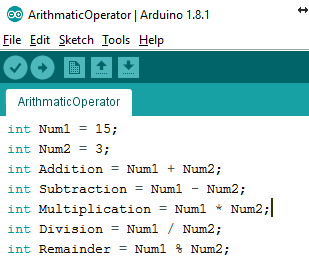# Arduino IDE: Arithmetic OperatorsDescription
Learn how to perform basic arithmetic operations with Arduino IDE, including addition, subtraction, multiplication, division, and remainder. Understand how data types affect the results of calculations and how to use the different arithmetic operators.

## Introduction

Arduino IDE is capable of doing basic arithmetic operations. Arduino has the following five arithmetic operators for basic mathematical operations:

OperatorsOperator SymbolExample
Addition+ a = b + 2017
Subtraction-b = 2017 - a
Multiplication*d = 2.5 * e
Division/e = d / 2.5
Remainder%f = d % 2.5

The addition operator is used for adding two numbers. Here is an example:

``````int Num1 = 15;
int Num2 = 3;
int Sum;

void setup(){
Serial.begin(9600);
Sum = Num1 + Num2;
Serial.print("Addition of Num1 and Num2 is ");
Serial.println(Sum);
}

void loop() {
}``````

Serial Monitor Response: `Addition of Num1 and Num2 is 18`

In the code above, two variables are defined, namely Num1 and Num2. A value is assigned to both of them as well. The third variable, i.e. Sum is defined and no value is assigned to it; hence, it contains a random number. This variable is used to store the sum of  Num1 and Num2; this value will overwrite the random value when you assign it to Sum. After statement 5 is executed, the Sum will have a value of 18.

## Subtraction

The subtraction operator subtracts one number from another. It is represented by the plus sign (+). Given below is an example:

``````int Num1 = 15;
int Num2 = 3;
int Result;

void setup(){
Serial.begin(9600);
Result = Num1 - Num2;
Serial.print("Subtraction of Num1 and Num2 is ");
Serial.println(Result);
}

void loop() {
}``````

Serial Monitor Response: `Subtraction of Num1 and Num2 is 12`

The result of this operation is 12.

## Multiplication

The multiplication operator multiplies one number with another. It is represented by the asterisk (*). Given below is an example:

``````int Num1 = 15;
int Num2 = 3;
int Result;

void setup(){
Serial.begin(9600);
Result = Num1 * Num2;
Serial.print("Multiplication of Num1 and Num2 is ");
Serial.println(Result);
}

void loop() {
}``````

Serial Monitor Response: `Multiplication of Num1 and Num2 is 15`

The result of this operation is 45.

## Division

The division operator divides one number by another. It is represented by the division sign (/). Given below is an example:

``````int Num1 = 15;
int Num2 = 3;
int Result;

void setup(){
Serial.begin(9600);
Result = Num1 / Num2;
Serial.print("Division of Num1 and Num2 is ");
Serial.println(Result);
}

void loop() {
}``````

Serial Monitor Response: `Division of Num1 and Num2 is 5`

The result of this operation is 5.

There is, however, one more thing you should know about division. Till now we have used division only for integers and have got an integer as a result. But what if the result is a floating-point number (numbers with a decimal) and not an integer? Let’s find out with the help of the example given below:

``````int Num1 = 6;
int Num2 = 5;
int Result;

void setup(){
Serial.begin(9600);
Result = Num1 / Num2;
Serial.print("Division of Num1 and Num2 is ");
Serial.println(Result);
}

void loop() {
}``````

Serial Monitor Response: `Division of Num1 and Num2 is 1`

The result will be 1 because the numbers after the decimal point are discarded when the result is stored in the variable because its data type is int. However, if we use float as the data type of the variable to store the result, we get the correct result.

``````int Num1 = 6;
int Num2 = 5;
float Result;

void setup(){
Serial.begin(9600);
Result = (float) Num1 / Num2;
Serial.print("Division of Num1 and Num2 is ");
Serial.println(Result);
}

void loop() {
}``````

Serial Monitor Response: `Division of Num1 and Num2 is 1.20`When using constant values in calculations that store the result in a floating point variable, use a decimal point and a zero for whole numbers, e.g. 5.0 instead of 5.

## Remainder

The remainder operator calculates the remainder after one number is divided by another number. It is represented by the percentage sign (%). Given below is an example:

``````int Num1 = 6;
int Num2 = 5;
int Result;

void setup(){
Serial.begin(9600);
Result = Num1 % Num2;
Serial.print("Remainder of Num1 and Num2 is ");
Serial.println(Result);
}

void loop() {
}``````

Serial Monitor Response: `Remainder of Num1 and Num2 is 1`

The result of this operation is 1.

## Conclusion

In conclusion, Arduino IDE is capable of performing basic arithmetic operations such as addition, subtraction, multiplication, division, and remainder. The arithmetic operators used in Arduino are + for addition, – for subtraction, * for multiplication, / for division, and % for remainder. The data type of the result of a mathematical operation should be taken into consideration when assigning the result to a variable. The float should be used if the result is a decimal number.